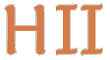# Hilbert II

 English Deutsch
introduction
news
mathematics
QEDEQ
planning
glossary
development
prototype
installation
manual
propositional calculus
predicate calculus
QEDEQ
 module formula QEDEQ text
problems
contact
site map
Prototype / PMII QEDEQ Module / BNF text description

Here is the description of a QEDEQ text module in BNF notation. For an example text file see: Axiomatic Set Theory (at least it is close to this definition).

```<qedeq module> ::= <header>
<paragraphs>

<header>       ::= <spec>               % specifies this qedeq module
<title>
<abstract>           % describes contents
Module admin: <email>% email to this address if you want to inform the
%  this module)
<authors>
[<imports>]          % import these qedeq modules
[<usedby>]           % these qedeq modules use this module

<spec>         ::= Qedeq specification:
Name:      <name>    % name of this module, must be identical
%  with first part of file name
Version:   <version> % must be identical with second part
%  of file name
Rules:     <version> % this version of pmii is needed for
%  checking the correctness of this file
Locations: {<location>} % web URL of this qedeq module

<authors>      ::= Author(s): {<author> [(<email>)]}

<imports>      ::=  Needs the following qedeq modules:
{import}

<import>       ::=  <spec>               % now relative URLs are ok to describe a location
<label>              % alias, label prefix to use for that module

<paragraphs>   ::= {<paragraph>}

<paragraph>    ::= "(" <label> ")"       % for referencing
[<latex>]             % preceding text

(<abbreviation> | <axiom> | <declaration> | <definition> | <proposition>)

[<latex>]             % following text

<abbreviation> ::= <formula> := <formula> % first formula has pattern variables as arguments
% in the second formula the same pattern variables
% must occur

<declaration>  ::= <name>                % name of rule to declare
<description>         % short description of rule
{<link>}              % reference(s) to needed axioms or propositions

<axiom>        ::= <formula>             % this is an axiom

<proposition>  ::= <sentence>
<proof>

<sentence>     ::= <formula>             % this formula could be proved, no pattern
%  variable allowed

<proof>        ::= {<line>}              % last proof line formula must be equal to sentence
%  formula

<line>         ::= <formula>             % formula that could be deduced by previous proof lines
% or axioms, abbreviations, definitions or declared rules
{<lineref>}